##全国校区# Python办公自动化｜自动更新不对称表格2020-09-28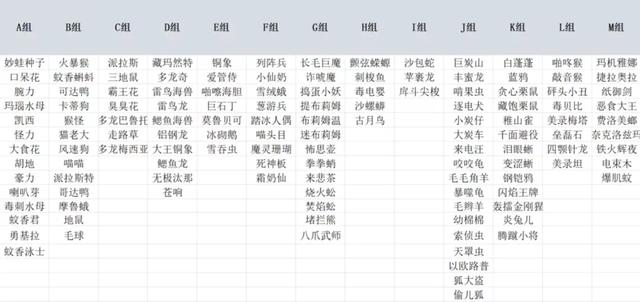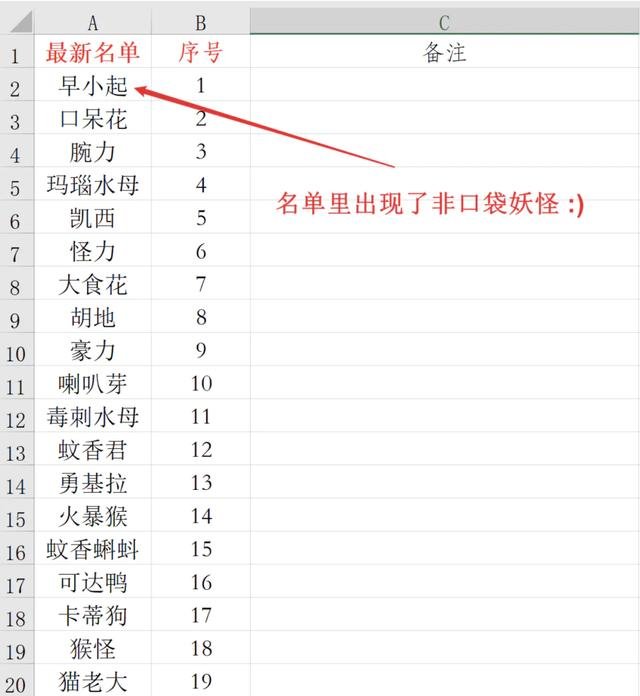Python实现

```import os
import pandas as pd
import numpy as np

def GetDesktopPath():
return os.path.join(os.path.expanduser("~"), 'Desktop')

path = GetDesktopPath() + '\\data\\'```

```df1 = pd.read_excel(path + '总名单.xlsx',encoding = 'utf-8',sheet_name = 0,skiprows=1)
df2 = df1.iloc[:,1:23]

df3 = pd.read_excel(path + '新名单.xlsx',encoding = 'utf-8',sheet_name = 0)```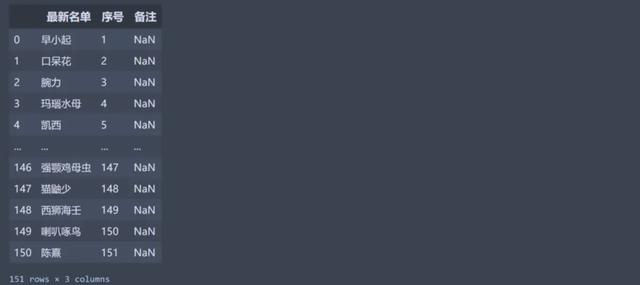```np.where(df2 == '死神板')
# (array(, dtype=int64), array(, dtype=int64))```

```col = np.where(df2 == '死神板')
df2.columns[col]
# 'F组'```

```def find(x):
results = np.where(df2 == x)
try:
return df2.columns[results]
except:
return '未分组'

df3['备注'] = df3['最新名单'].apply(find)```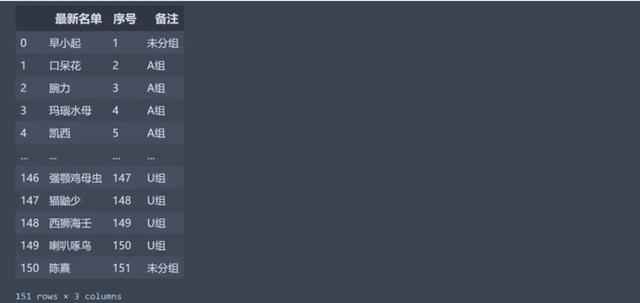```results_lst = []
for index,i in enumerate(df2.columns):
results = df3.iloc[np.where(df3['备注']==i).tolist(),0]
# 重置索引很重要，为什么重要往下看
results = results.reset_index(drop=True)
results_lst.append(results)
results_lst```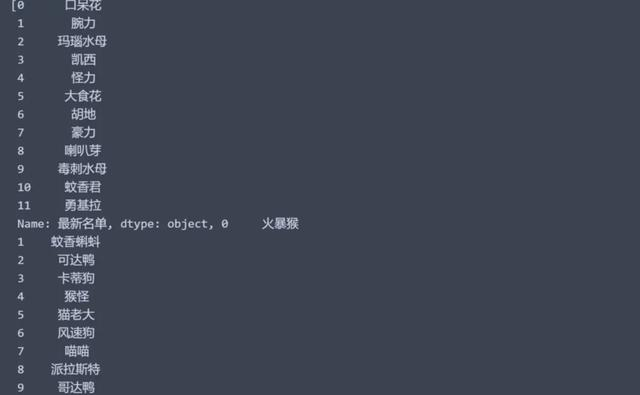```df_final = pd.concat(results_lst,axis=1)
# 记得把列名还原
df_final.columns = df2.columns```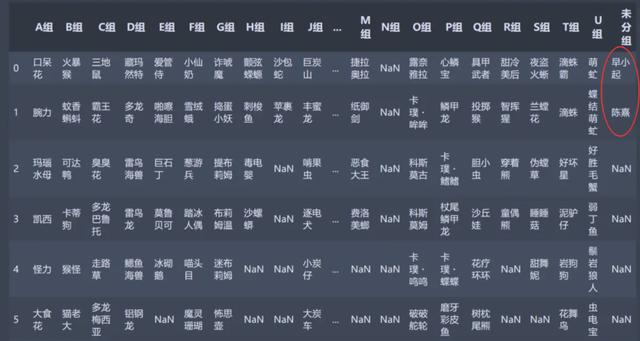```df_final.to_excel(f'{path}整理后表格.xlsx',
encoding='gbk', # 编码不一定是gbk
index=False,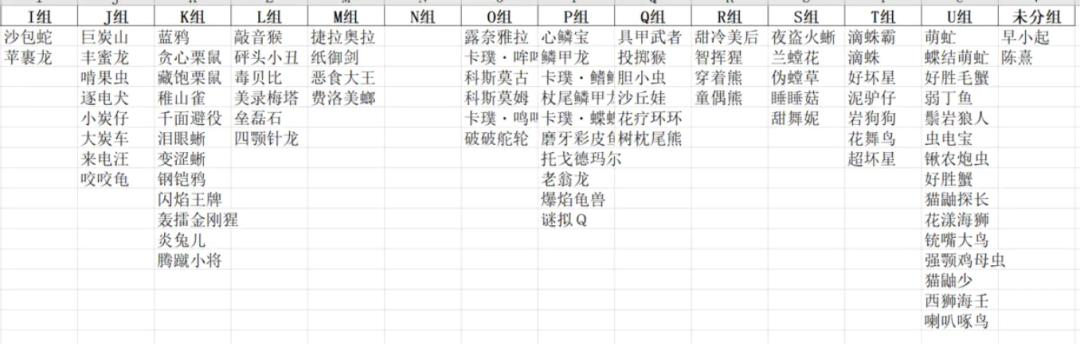OK